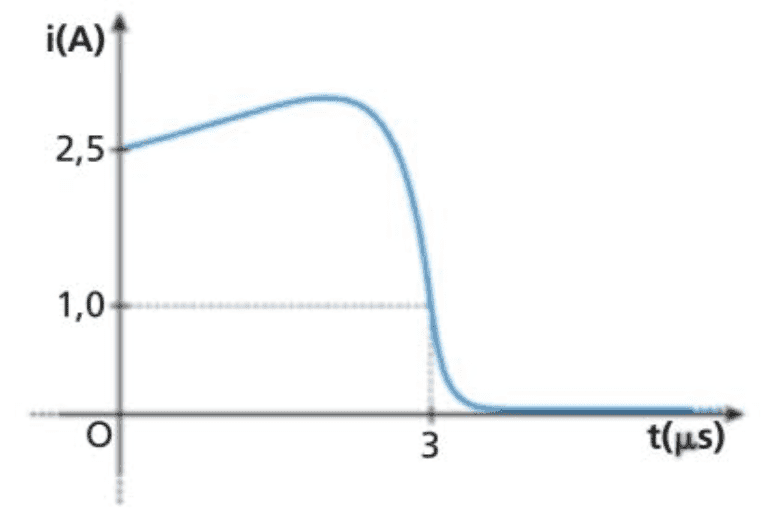# Question about a simple model for for back EMF

FranzDiCoccio
Summary:: I've found an exercise about sparks caused by back emf. The exercise is based on a mathematical model of the current flowing in the circuit around the time when the switch is closed.
I'm wondering to what extend that model is realistic

The exercise I'm referring to proposes this function for the current
$$i(t) = \left\{ \begin{array}{ll} \displaystyle\frac{t^2+a t + b}{(t-4)^2} & 0\leq t \leq 3 \\ e^{-c(t-3)} & t>3 \end{array} \right.$$
where the parameters are such that the graph of the function isThen the problem gives the self-inductance of the circuit and the breakdown voltage, and asks for the time at which the spark happens.

The problem is not too hard, per se.

I'm wondering: is the given function any realistic? If so, is there any simple argument that explains why?

The only thing I can think of is that the given function is a smoothed out "heuristic" version of the two functions describing the charging and the discharging of an inductor. The first part grows as ## i_{\rm max} (1-e^{-t/\tau})##, the second decreases as ## i_{\rm max} e^{-t/\tau}##.

The other possibility is that the given function is not realistic and was devised for the only purpose of making this exercise solvable.

Thanks a lot for any insight

•Delta2

Homework Helper
Gold Member
Did you mean to say "when the switch is opened"? If not, what kind of circuit is this in which the current goes to zero when the switch is closed?

FranzDiCoccio
Did you mean to say "when the switch is opened"? If not, what kind of circuit is this in which the current goes to zero when the switch is closed?
Sorry, yes. I do mean "when the switch is opened". I always get that backwards. Apologies.
The current is flowing in the circuit, and then the switch is opened, causing the current to vanish.

I tried editing the summary but it does not seem to be possible.

Mentor
I've found an exercise about sparks caused by back emf.
Is there a schematic that goes with this problem? I need some visuals...FranzDiCoccio
no, sorry. I think the problem is quite generic. That's why I'm asking. I tried a few web searches, to no avail. Perhaps I used the wrong keywords.

The text refers to a (possibly very simple) circuit with some self-inductance. The current flowing inside that is direct, from what I understand.
The solver is supposed to apply Faraday law and find the time when the induced emf becomes larger than the breakdown potential.
That is: the problem is mostly mathematical. That's why I was wondering whether there is some more robust justification for the given function.

I would also be happy to know whether there are (simple) models for this kind of problems. Not necessarily the exactly same function, but perhaps something similar. I suppose the main features would be the same: the function slightly grows until the switch is opened, at which point it starts to decrease and would eventually go to zero, except a spark could happen sometimes before it vanishes.
This is because the decrease of the function is pretty steep.

I guess one could start with a very naive model where the ##i(t) = i_{\rm max}(1-e^{-t/\tau})## before the switch-off time ##t_0## and ##i(t)=i(t_0)e^{-(t-t_0)/\tau}## after that. The induced emf would not be continuous, though.
The given function is devised such that its derivative is at least continuous. It has a cusp at t=3. Its second derivative is not continuous, but this is not very important for the exercise.

Homework Helper
Gold Member
I don't think that the part of function for ##0\leq t\leq 3## is realistic as the current is the ratio of two polynomials of second degree. You need a digital circuit to produce such current i doubt it can be achieved by only using R,L,C as elements.

•alan123hk
FranzDiCoccio
so in a "simple" circuit the problem would be solved simply considering the induced emf after opening the switch
$$V(t) =L \frac{i(t_0)}{\tau} e^{-t/\tau}$$
and checking if and when it is larger than the breakdown potential?

alan123hk
•Delta2
Homework Helper
Gold Member
It would also be useful to have some idea of the inductance ## L ## of the circuit. If it is even on the order of 1-10 mH, you can see with ## \mathcal{E}=-L \frac{di}{dt} ##, that you can generate some hefty voltages by having the current drop an ampere or two in less than one microsecond.

•Delta2